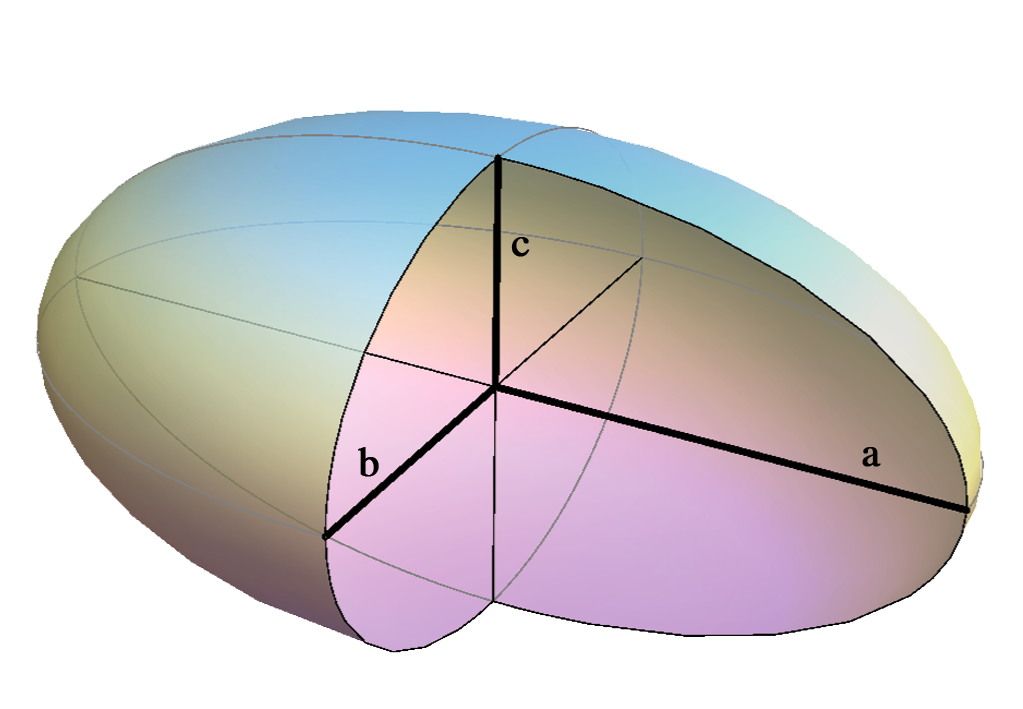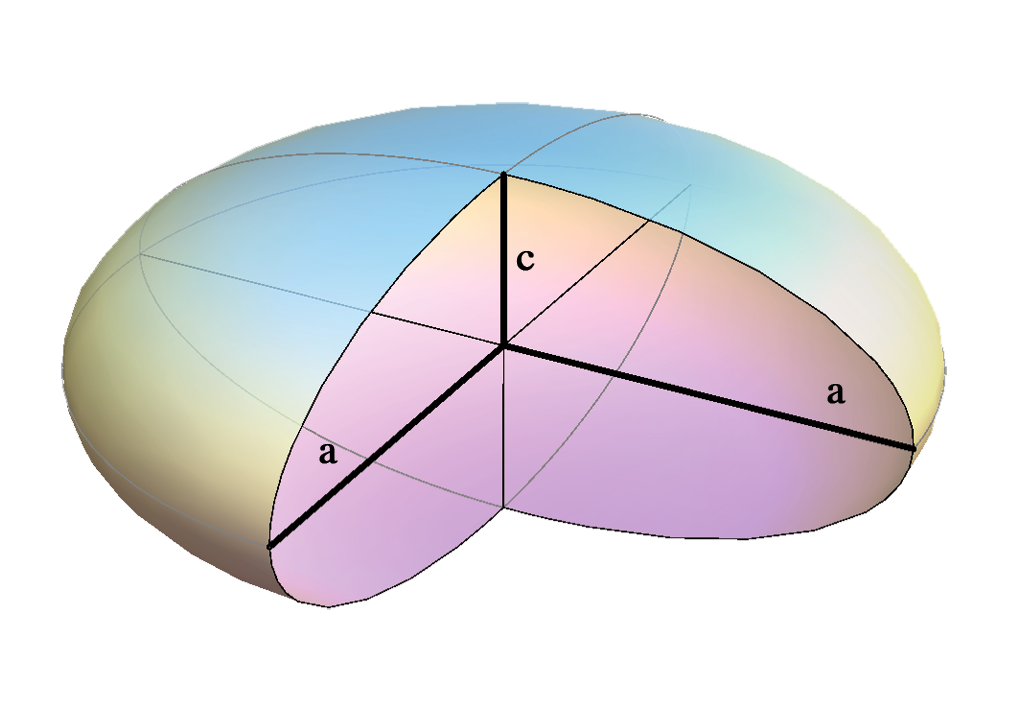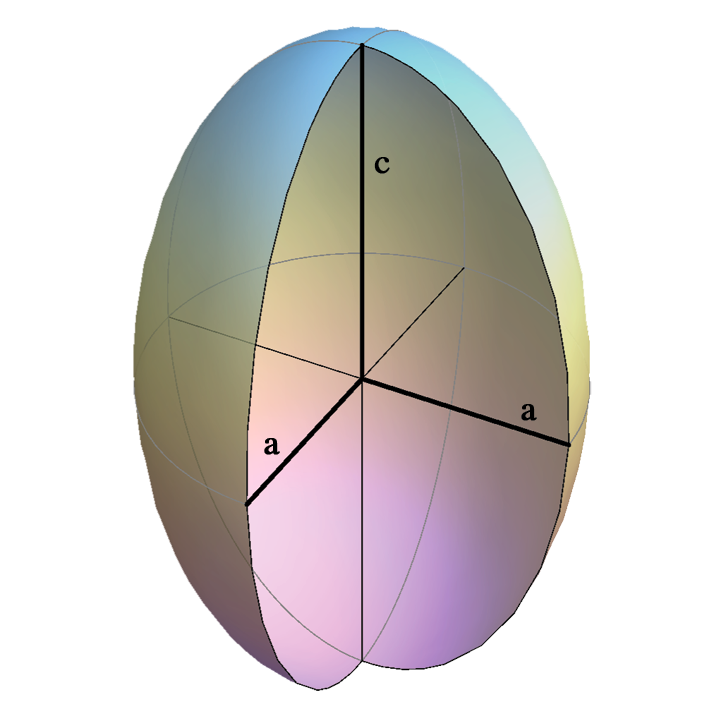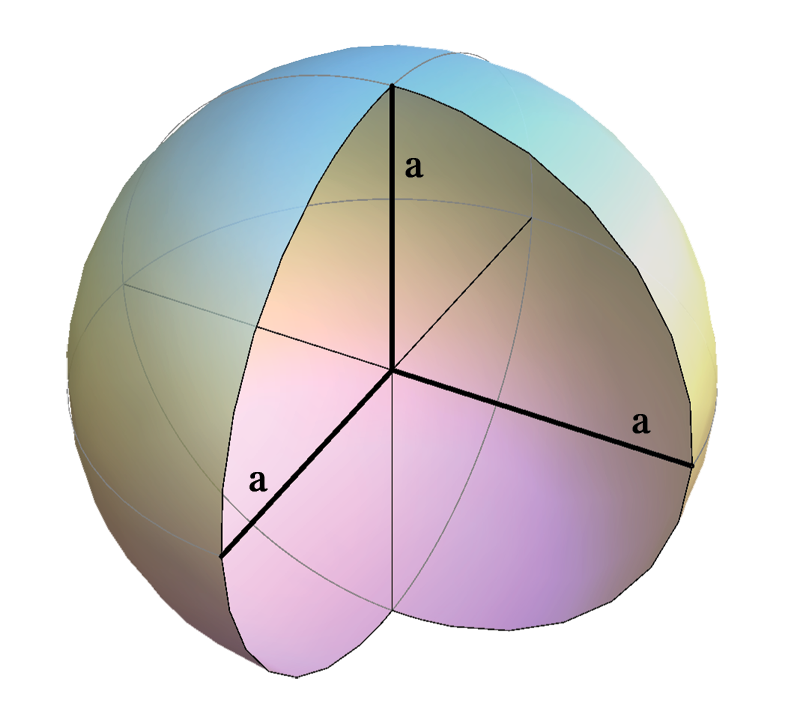## Ellipsoids

 A tri-axial ellipsoid $$\frac{x^2}{a^2}+\frac{y^2}{b^2}+\frac{z^2}{c^2}=1$$ Real plane intersections are the ellipses and the circles.An ellipsoid of revolution $$\frac{x^2}{a^2}+\frac{y^2}{a^2}+\frac{z^2}{c^2}=1$$ Real plane intersections are the ellipses and the circles. $$a > c$$ an oblate ellipsoidobtained by rotating an ellipse about its minor axis right-click $$\rightarrow$$ PLAY $$a < c$$ an prolate ellipsoidobtained by rotating an ellipse about its major axis right-click  $$\rightarrow$$ PLAY
 a sphere $$x^2+y^2+z^2=a^2$$ Real plane intersection is a circle.DOMES (architecture)

Created by Sonja Gorjanc, translated by Helena Halas and Iva Kodrnja - 3DGeomTeh - Developing project of the University of Zagreb